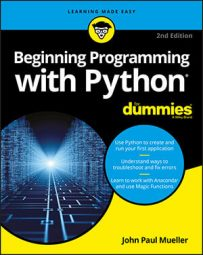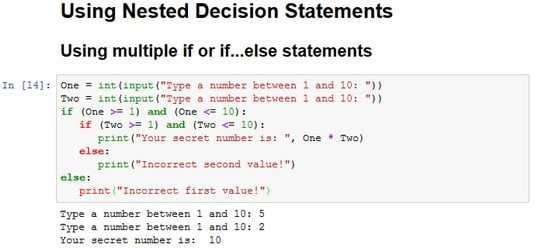##### Beginning Programming with Python For DummiesThe decision-making process often happens in levels, both in real life and in Python programming. For example, when you go to the restaurant and choose eggs for breakfast, you have made a first-level decision. Now the server asks you what type of toast you want with your eggs. The server wouldn’t ask this question if you had ordered pancakes, so the selection of toast becomes a second-level decision. When the breakfast arrives, you decide whether you want to use jelly on your toast. This is a third-level decision. If you had selected a kind of toast that doesn’t work well with jelly, you might not have had to make this decision at all.

This process of making decisions in levels, with each level reliant on the decision made at the previous level, is called nesting. Developers often use nesting techniques to create applications that can make complex decisions based on various inputs. The information here describes several kinds of nesting you can use within Python to make complex decisions.

## Using multiple if or if…else statements

The most commonly used multiple selection technique is a combination of `if` and `if…else` statements. This form of selection is often called a selection tree because of its resemblance to the branches of a tree. In this case, you follow a particular path to obtain a desired result. The following steps show how to create a selection tree:
1. Type the following code into the notebook — pressing Enter after each line:

`One = int(input("Type a number between 1 and 10: "))` `Two = int(input("Type a number between 1 and 10: "))```` if (One >= 1) and (One <= 10):``` `if (Two >= 1) and (Two <= 10):` `print("Your secret number is: ", One * Two)` `else:` `print("Incorrect second value!")` `else:` `print("Incorrect first value!")`

Notice, the second `if…else` statement is indented within the first if…else statement. The indentation tells Python that this is a second-level statement.

2. Click Run Cell.

You see a Python Shell window open with a prompt to type a number between 1 and 10.

3. Type 5 and press Enter.

The shell asks for another number between 1 and 10.

4. Type 2 and press Enter.

You see the combination of the two numbers as output.Adding multiple levels lets you perform tasks with greater complexity.

If you attempt to provide a value that’s outside the requested range, you see an error message. The error message is tailored for either the first or second input value so that the user knows which value was incorrect.

Providing specific error messages is always useful because users tend to become confused and frustrated otherwise. In addition, a specific error message helps you find errors in your application much faster.

## Combining other types of decisions

You can use any combination of `if`, `if…else`, and `if…elif` statements to produce a desired outcome. You can nest the code blocks as many levels deep as needed to perform the required checks. For example, this code shows what you might accomplish for a breakfast menu.

`print("1. Eggs")`

`print("2. Pancakes")`

`print("3. Waffles")`

`print("4. Oatmeal")`

`MainChoice = int(input("Choose a breakfast item: "))`

`if (MainChoice == 2):`

`Meal = "Pancakes"`

`elif (MainChoice == 3):`

`Meal = "Waffles"`

`if (MainChoice == 1):`

`print("1. Wheat Toast")`

`print("2. Sour Dough")`

`print("3. Rye Toast")`

`print("4. Pancakes")`

`Bread = int(input("Choose a type of bread: "))`

`if (Bread == 1):`

`print("You chose eggs with wheat toast.")`

`elif (Bread == 2):`

`print("You chose eggs with sour dough.")`

`elif (Bread == 3):`

`print("You chose eggs with rye toast.")`

`elif (Bread == 4):`

`print("You chose eggs with pancakes.")`

`else:`

`print("We have eggs, but not that kind of bread.")`

`elif (MainChoice == 2) or (MainChoice == 3):`

`print("1. Syrup")`

`print("2. Strawberries")`

`print("3. Powdered Sugar")`

`Topping = int(input("Choose a topping: "))`

`if (Topping == 1):`

`print ("You chose " + Meal + " with syrup.")`

`elif (Topping == 2):`

`print ("You chose " + Meal + " with strawberries.")`

`elif (Topping == 3):`

`print ("You chose " + Meal + " with powdered sugar.")`

`else:`

`print ("We have " + Meal + ", but not that topping.")`

`elif (MainChoice == 4):`

`print("You chose oatmeal.")`

`else:`

`print("We don't serve that breakfast item!")`

This example has some interesting features. For one thing, you might assume that an `if…elif` statement always requires an `else` clause. This example shows a situation that doesn’t require such a clause. You use an `if…elif` statement to ensure that Meal contains the correct value, but you have no other options to consider.

The selection technique is the same as you saw for the previous examples. A user enters a number in the correct range to obtain a desired result. Three of the selections require a secondary choice, so you see the menu for that choice. For example, when ordering eggs, it isn’t necessary to choose a topping, but you do want a topping for pancakes or waffles.

Notice that this example also combines variables and text in a specific way. Because a topping can apply equally to waffles or pancakes, you need some method for defining precisely which meal is being served as part of the output. The `Meal` variable that the application defines earlier is used as part of the output after the topping choice is made.

The best way to understand this example is to play with it. Try various menu combinations to see how the application works.